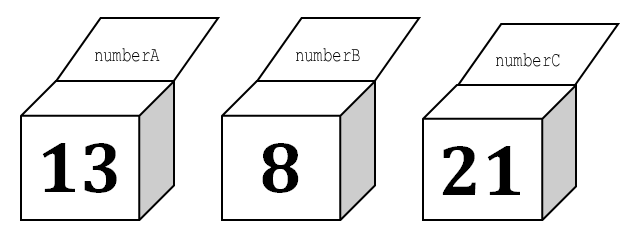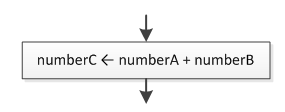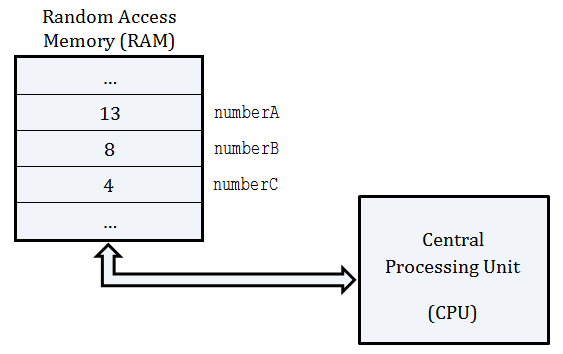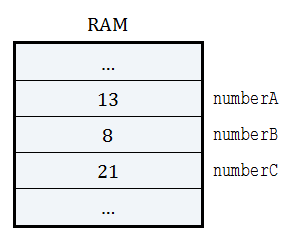In computer science, a variable is a location in the computer’s main memory (RAM) where you can store a value and change it as the program executes.

Picture a variable as a transparent box in which you can insert and hold one thing at a time. Because the box is transparent, you can also examine what it contains. Moreover, if you have two or more boxes you can give each box a unique name. For example, you could have three boxes, each containing a different number, and you could name the boxes` numberA, numberB, and numberC.`In a flowchart, the action of storing a value in a variable is represented by a left arrowThis action is usually expressed as “Assign a value, or the result of an expression, to a variable.” The left arrow is called the “value assignment operator.”

Notice: Please note that this arrow always points to the left. You are not allowed to use right arrows. Also, on the left side of the arrow only one single variable should exist.

In real computer science, the three boxes are actually three individual regions in main memory (RAM), named `numberA, numberB and numberC`.When a program instructs the CPU to execute the statement

`numberC ← numberA + numberB`

it follows the same three-step process as in the previous example.

1. The number 13 is transferred from the RAM’s region named `numberA` to the CPU. The number 8 is transferred from the RAM’s region named `numberB` to the CPU. (This is the first step, in which you examined the values contained in the first two boxes.)
1. The CPU calculates the sum of 13 + 8. (This is the second step, in which you used your brain to calculate the sum, or result.)
1. The result, 21, is transferred from the CPU to the RAM’s region named `numberC`, replacing the existing number 4. (This is the third step, in which you inserted the result in the last box.)

After execution, the RAM looks like this.Remember! While a program is running, a variable can hold various values, but only one value at a time. When you assign a value to a variable, this value remains stored until you assign a new value. The old value is lost.

Remember! A variable is one of the most important elements in computer science because it helps you interact with data stored in the main memory (RAM).